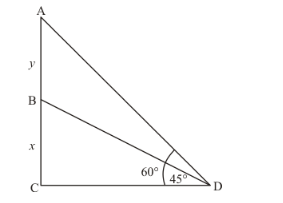# A vertical tower stands on a horizontal plane and is surmounted by a vertical flag-staff.

Question:

A vertical tower stands on a horizontal plane and is surmounted by a vertical flag-staff. At a point on the plane 70 metres away from the tower, an observer notices that the angles of elevation of the top and the bottom of the flagstaff are respectively 60° and 45°. Find the height of the flag-staff and that of the tower.

Solution:

Let BC be the tower of height m and AB be the flag staff of height y, 70 m away from the tower, makes an angle of elevation are 60° and 45° respectively from top and bottom of the flag staff.

Let AB = y m, BC = x m and CD = 70 m.

$\angle A D C=45^{\circ}$ and $\angle A D C=60^{\circ}$

So we use trigonometric ratios.In a triangle,

$\Rightarrow \quad \tan D=\frac{B C}{C D}$

$\Rightarrow \quad \tan 45^{\circ}=\frac{x}{70}$

$\Rightarrow \quad 1=\frac{70}{x}$

$\Rightarrow \quad x=70$

Again in a triangle,

$\Rightarrow \quad \tan D=\frac{A B+B C}{C D}$

$\Rightarrow \quad \tan 60^{\circ}=\frac{y+x}{70}$

$\Rightarrow \quad \sqrt{3}=\frac{y+70}{70}$

$\Rightarrow \quad 70 \sqrt{3}=70+y$

$\Rightarrow \quad y=70(\sqrt{3}-1)$

$\Rightarrow \quad y=51.24$